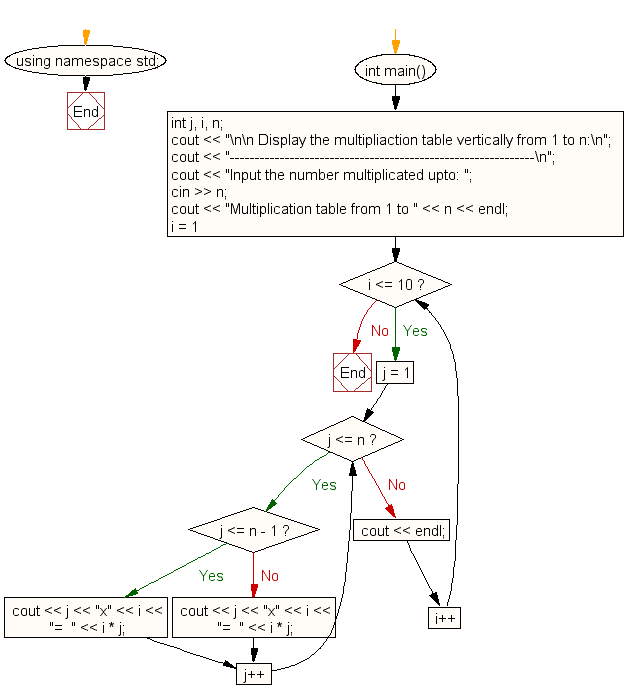﻿ C++ : Multiplication table vertically from 1 to n

# C++ Exercises: Display the multiplication table vertically from 1 to n

## C++ For Loop: Exercise-19 with Solution

Write a program in C++ to display the multiplication table vertically from 1 to n.

Sample Solution:-

C++ Code :

``````#include <iostream> // Include input-output stream header

using namespace std; // Using standard namespace to avoid writing std::

int main() // Start of the main function
{
int j, i, n; // Declaration of integer variables 'j' (loop counter), 'i' (loop counter), 'n' (user input for the limit of the multiplication table)

cout << "\n\n Display the multiplication table vertically from 1 to n:\n"; // Display message on the console
cout << "-------------------------------------------------------------\n";
cout << "Input the number up to 5: "; // Prompt the user to input the limit for the multiplication table
cin >> n; // Read the input value as the limit

cout << "Multiplication table from 1 to " << n << endl; // Display a message showing the range of the multiplication table

for (i = 1; i <= 10; i++) // Loop for numbers from 1 to 10 (rows of the table)
{
for (j = 1; j <= n; j++) // Nested loop for creating the multiplication table columns
{
if (j <= n - 1) // Checks if 'j' is less than the limit to print the table with proper formatting
cout << j << "x" << i << "=  " << i * j; // Display the product of 'i' and 'j' with proper formatting
else
cout << j << "x" << i << "=  " << i * j; // Display the product of 'i' and 'j' for the last column
}
cout << endl; // Move to the next line after printing each row of the table
}
}
``````

Sample Output:

``` Display the multipliaction table vertically from 1 to n:
-------------------------------------------------------------
Input the number multiplicated upto: 5
Multiplication table from 1 to 5
1x1=1  2x1=2  3x1=3  4x1=4  5x1=5
1x2=2  2x2=4  3x2=6  4x2=8  5x2=10
1x3=3  2x3=6  3x3=9  4x3=12  5x3=15
1x4=4  2x4=8  3x4=12  4x4=16  5x4=20
1x5=5  2x5=10  3x5=15  4x5=20  5x5=25
1x6=6  2x6=12  3x6=18  4x6=24  5x6=30
1x7=7  2x7=14  3x7=21  4x7=28  5x7=35
1x8=8  2x8=16  3x8=24  4x8=32  5x8=40
1x9=9  2x9=18  3x9=27  4x9=36  5x9=45
1x10=10  2x10=20  3x10=30  4x10=40  5x10=50
```

Flowchart:C++ Code Editor: